The first step in the design of an optical communication systems is to decide how the electrical signal should be converted into an bit stream. There are two typical choices for the modulation format of the signal:

• return-to-zero (RZ)
• nonreturn-to-zero (NRZ)

Examples of the modulation formats are shown in Figure 1.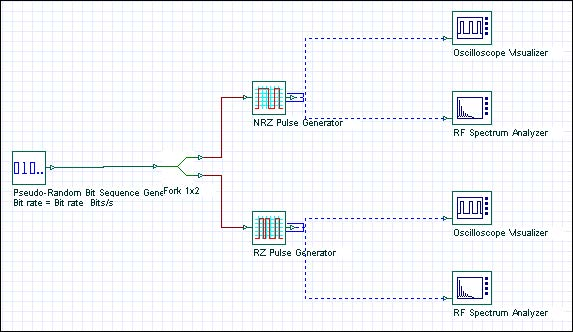Figure 1: Modulation Formats

In the RZ formats, each pulse representing bit 1 is shorter than the bit slot, and its amplitude returns to zero before the bit duration is over (see Figure 3). In the NRZ format, the pulse remains on throughout the bit slot and its amplitude does not drop to zero between two or more successive 1 bits (see Figure 2). As a result, pulse width varies depending on the bit pattern, whereas it remains the same in the case of RZ format.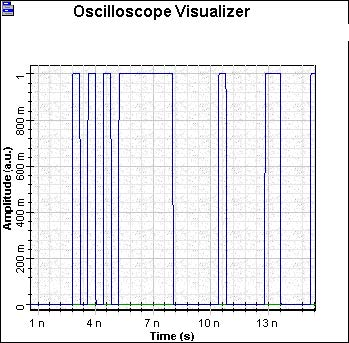Figure 2: NRZ – Time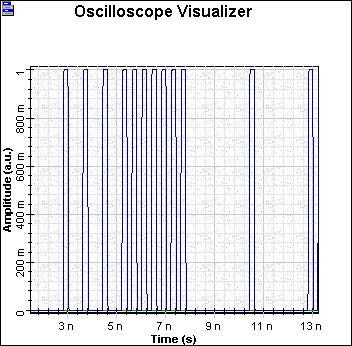Figure 3: RZ – Time

An advantage of the NRZ format is that the bandwidth associated with the bit stream is smaller than that of the RZ format by about a factor of 2, because on-off transitions occur fewer times (see Figure 4 and Figure 5).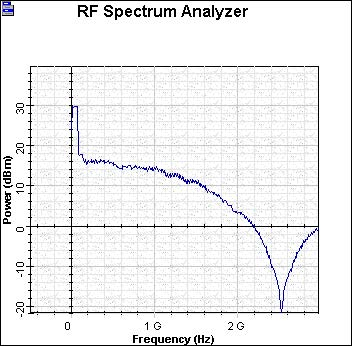Figure 4: NRZ – Frequency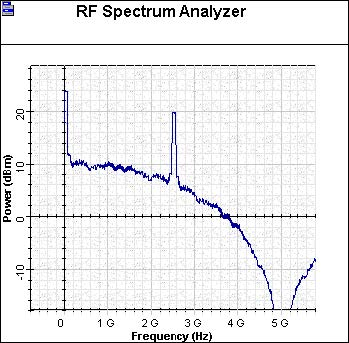Figure 5: RZ – Frequency